# Lab 1: Prisms

Our first chapter (Geometry: Learn About Shapes) has kids building various three-dimensional geometric shapes out of toothpicks and gumdrops*, drawing perfect circles, ellipses, and triangles with common household objects, and then drawing giant circles and ellipses outside.

* If you make a mistake, don't forget to eat the gumdrop to hide the evidence!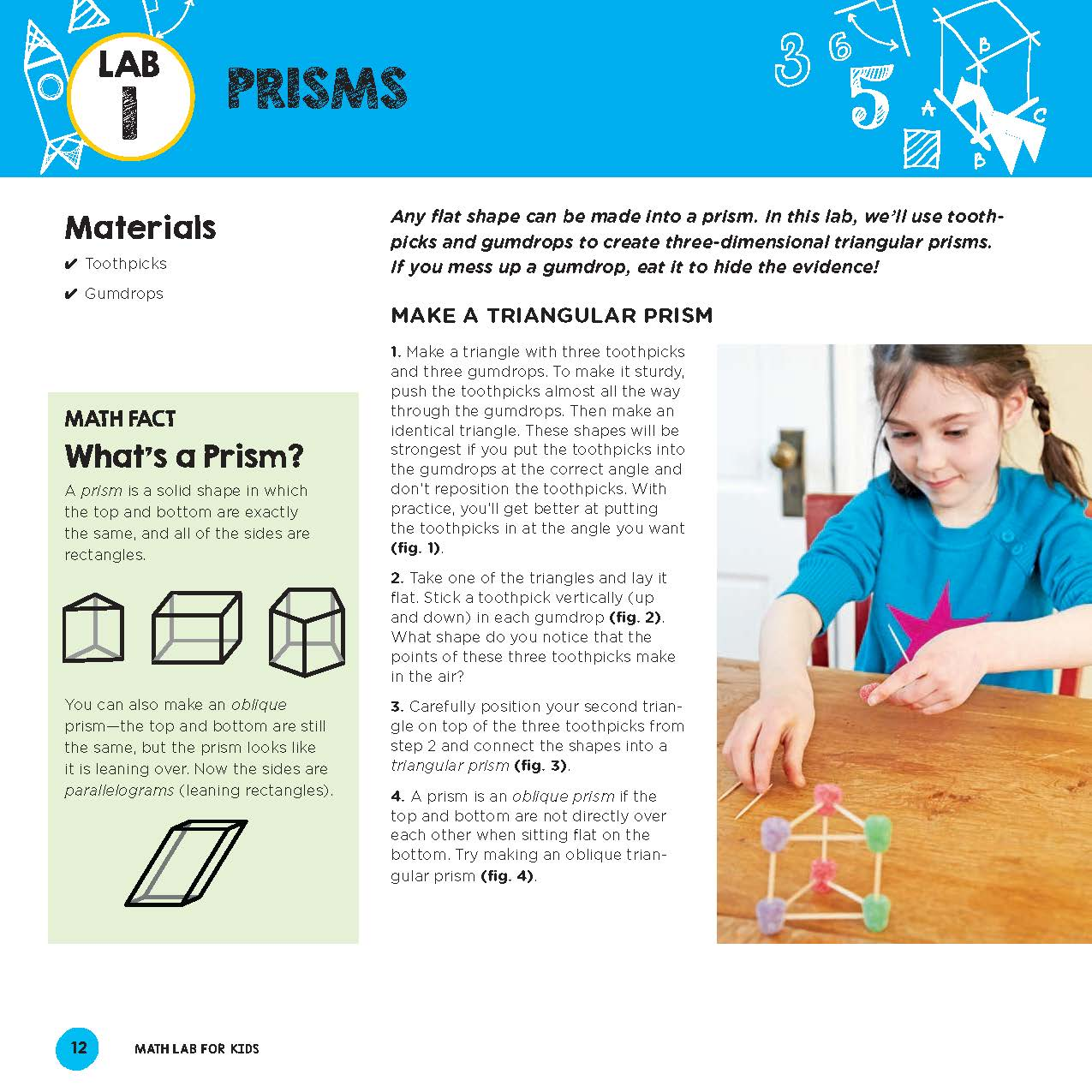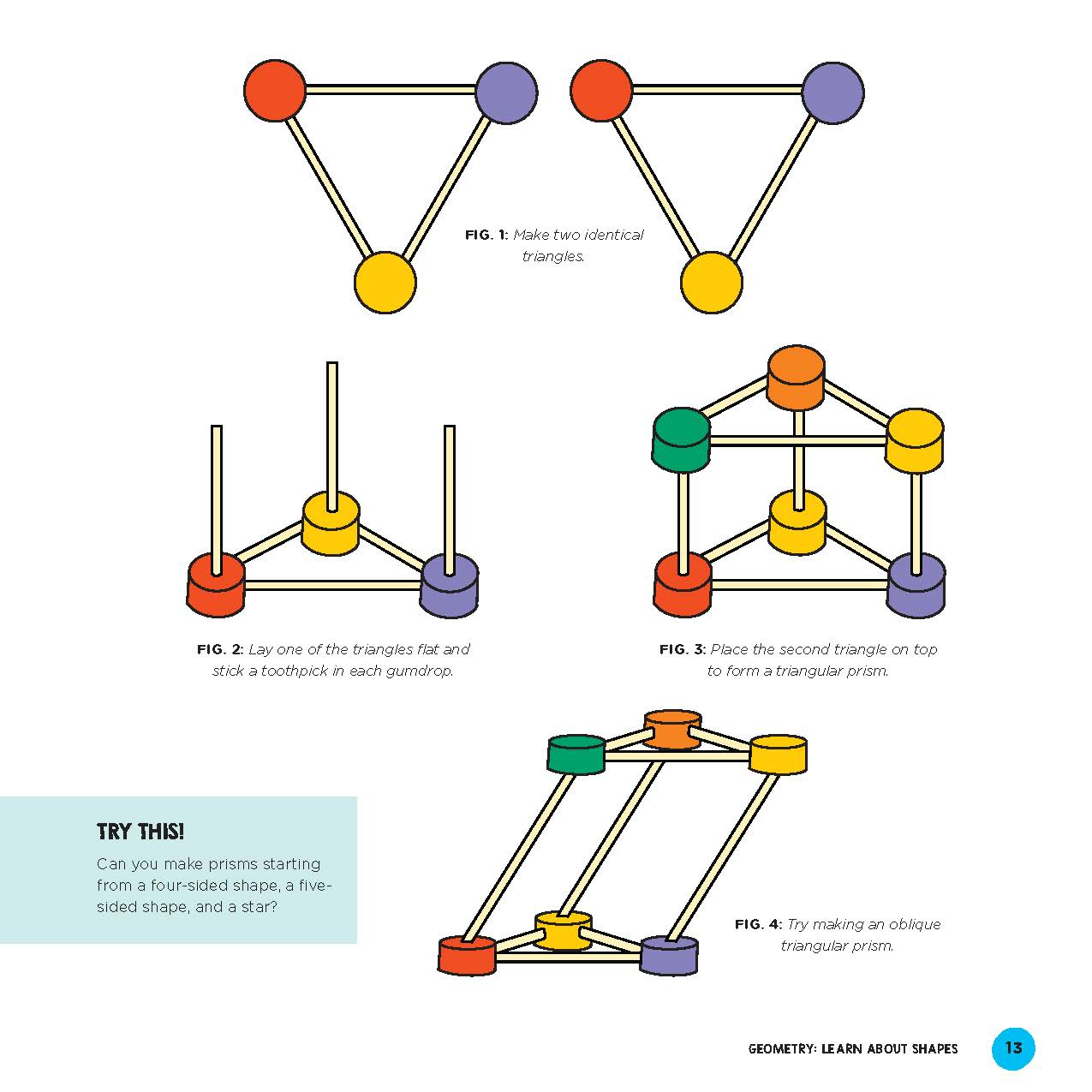Chapter 1 (Geometry: Learn About Shapes) labs are:

• Lab 1: Prisms
• Lab 2: Pyramids
• Lab 3: Antiprisms
• Lab 4: Platonic Solids
• Lab 5: Perfect Circles
• Lab 6: Try a Triangle
• Lab 7: Exact Ellipses
• Lab 8: Draw Giant Circles and Ellipses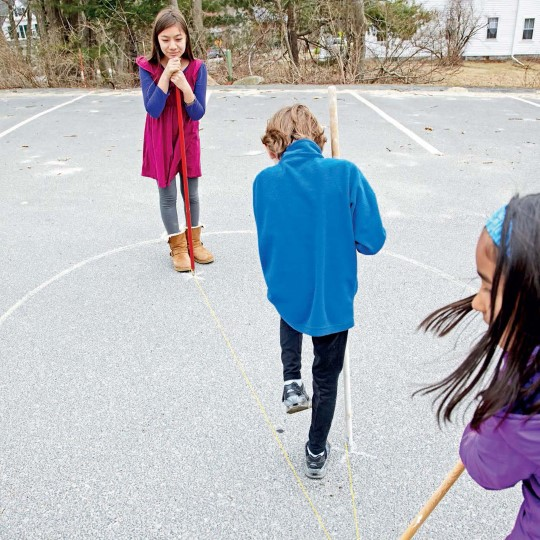# Lab 9: Compare and Classify Shapes

In Chapter 2 (Topology: Mind-Bending Shapes), kids compare and classify chapes, experiment with Möbius strips, and explore a Möbius surprise.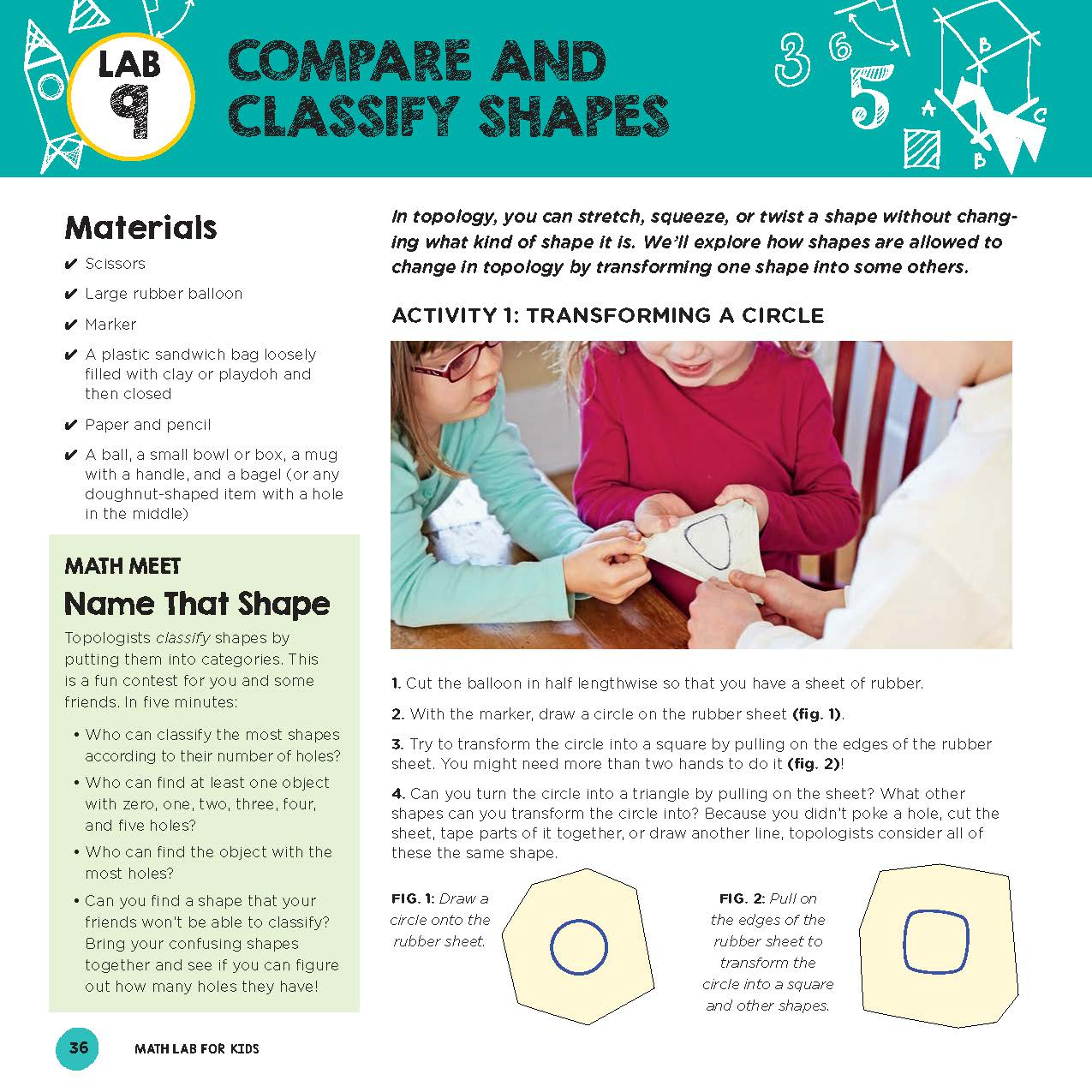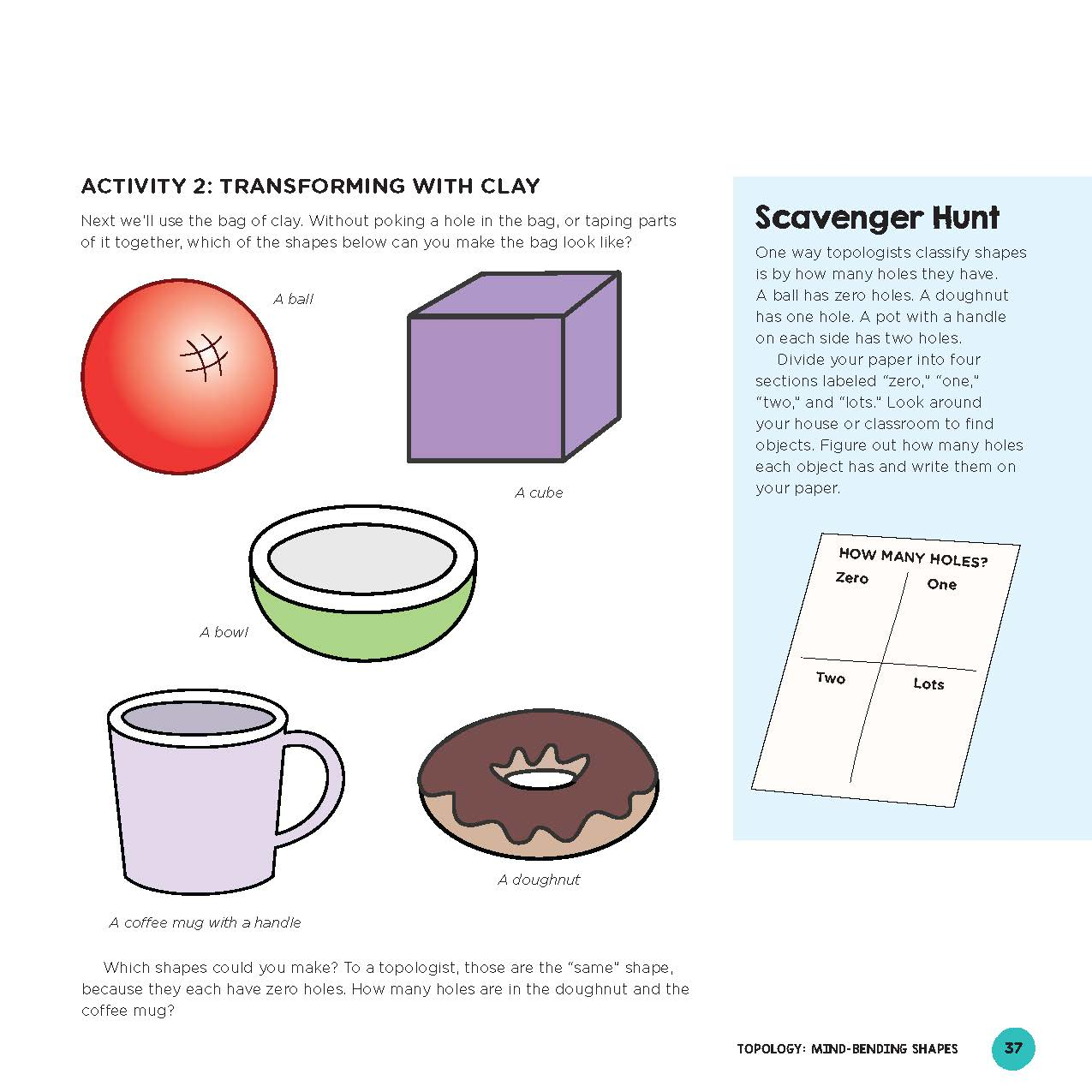Chapter 2 (Topology: Mind-Bending Shapes) labs are:

• Lab 9: Compare and Classify Shapes
• Lab 10: Möbius Strips
• Lab 11: Möbius Surprise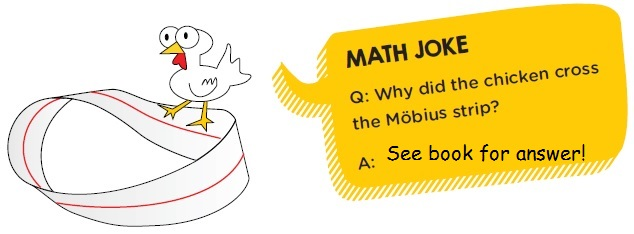# Lab 12: Map Coloring Basics

In Chapter 3, kids learn how to Color Maps like a Mathematician, a beautiful and surpisingly deep area of mathematics. Using the fewest number of colors possible, they fill in a map such that adjacent areas are different colors.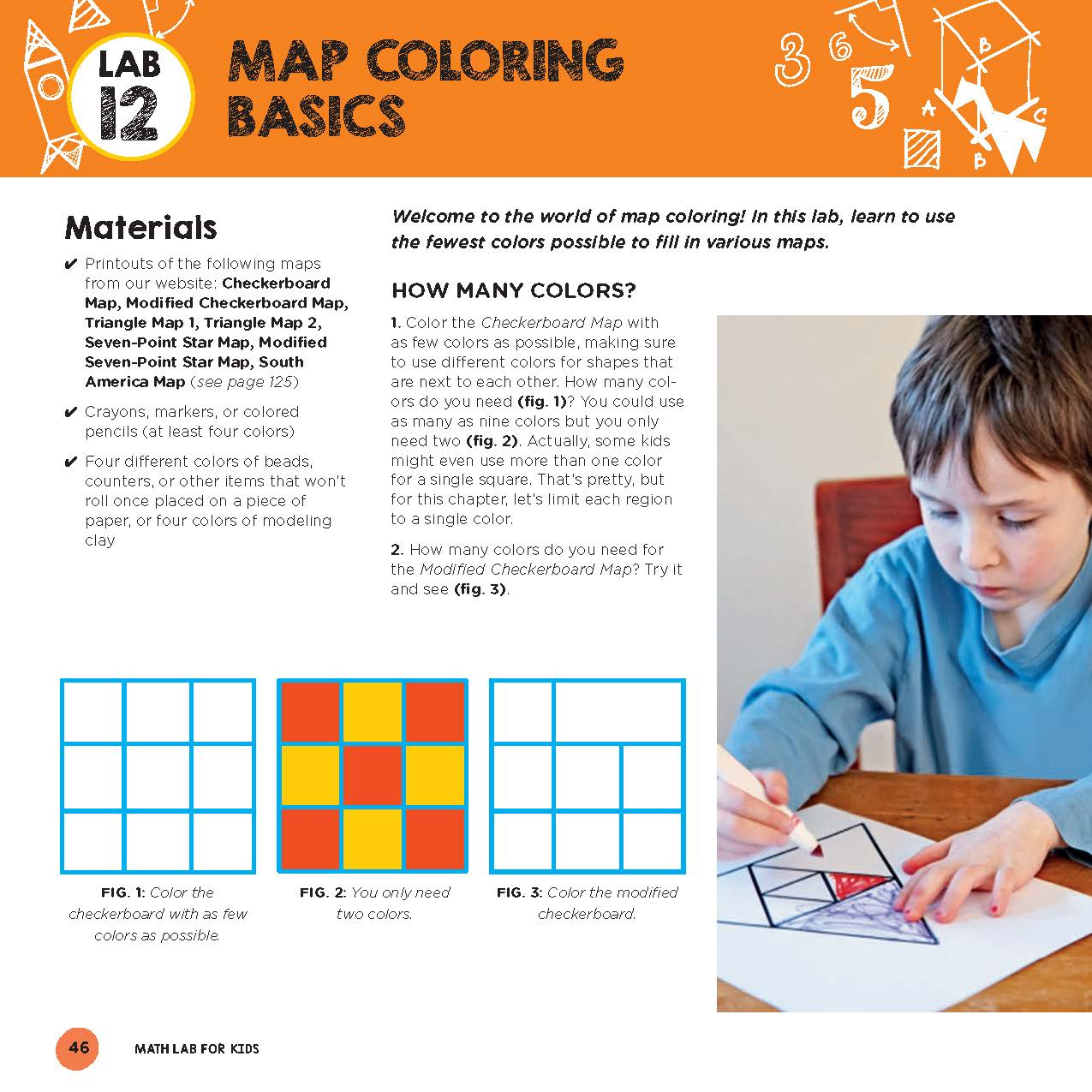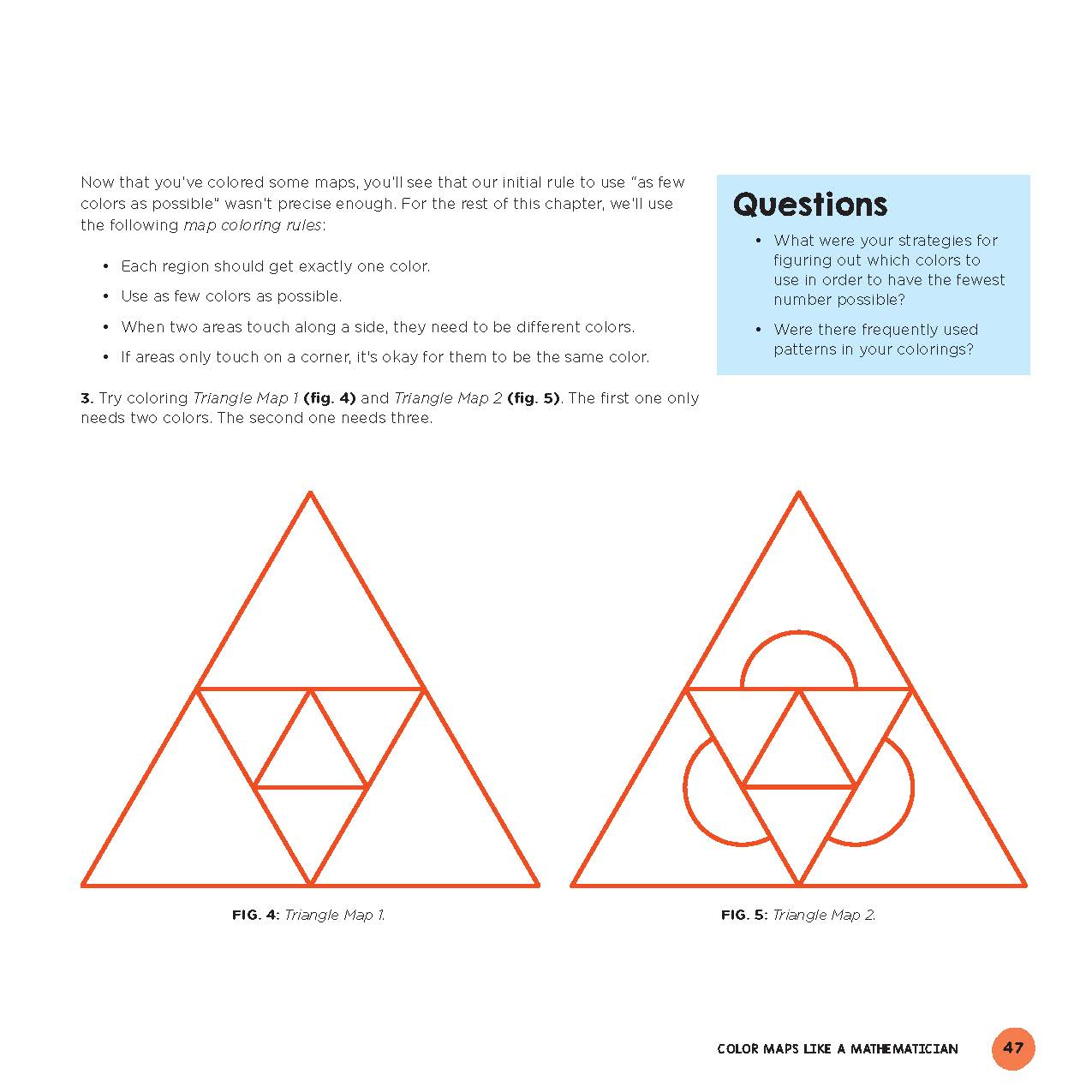Chapter 3, Color Maps like a Mathematician, labs are:

• Lab 12: Map Coloring Basics
• Lab 13: Efficient Map Coloring
• Lab 14: Squiggle Maps

## Happy Thanksgiving!

In lab 13, we color this turkey. (Truly a great coincidence!)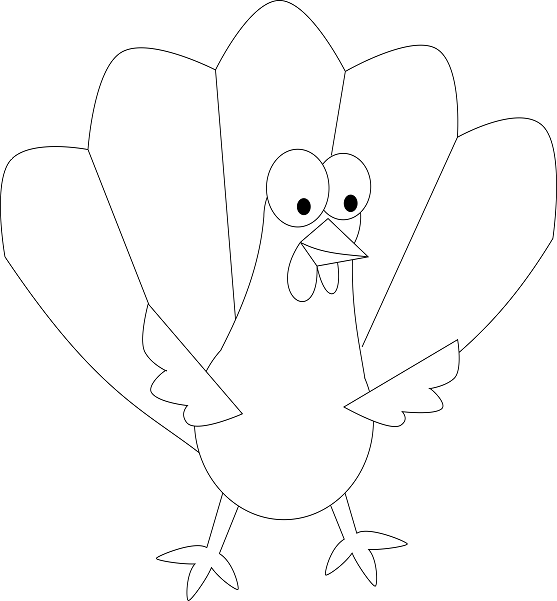# Lab 15: Drawing Parabolas

In Chapter 4, Stitching Curves, kids learn the art of "curve stitching," creating beautiful art while learning to approximate curves using straight lines.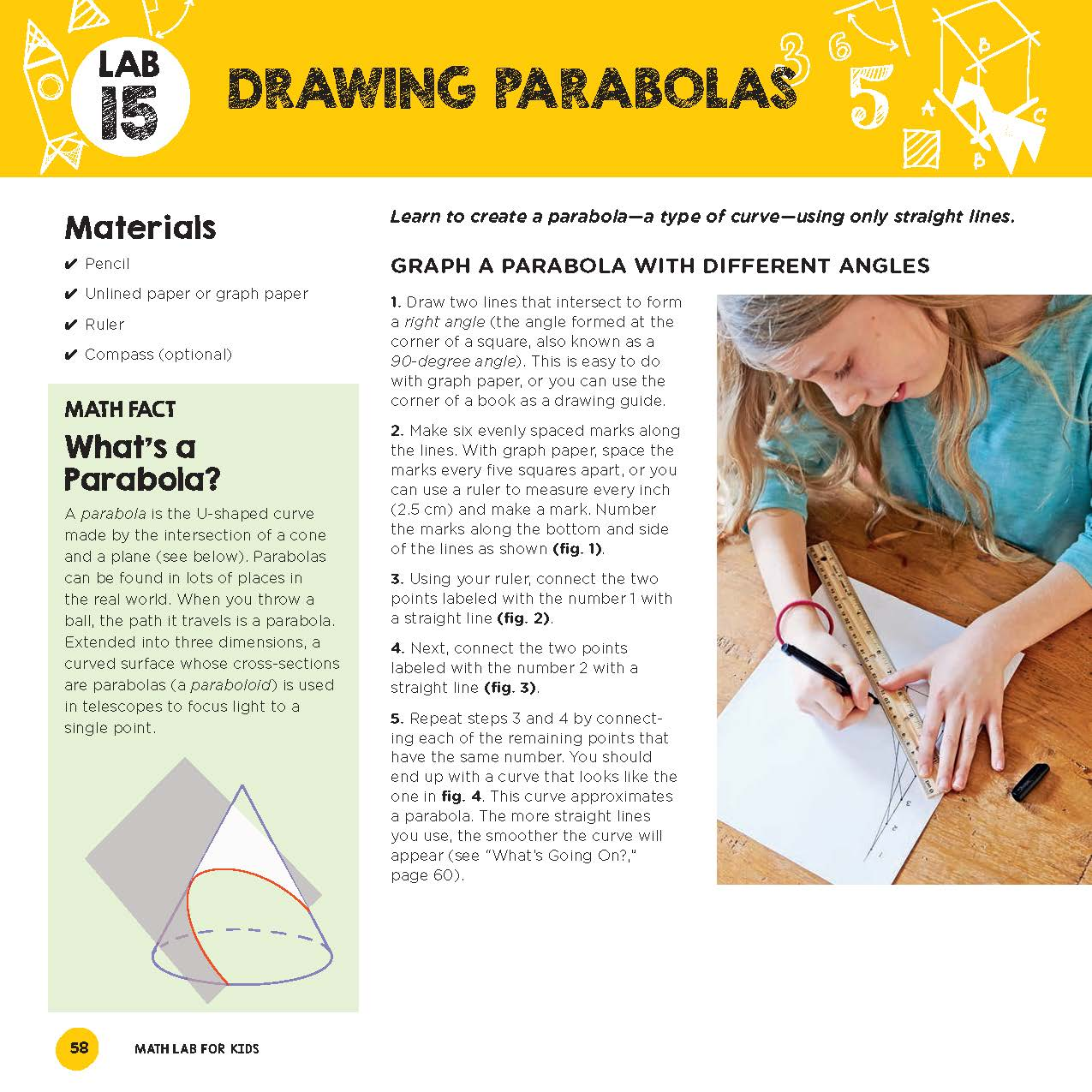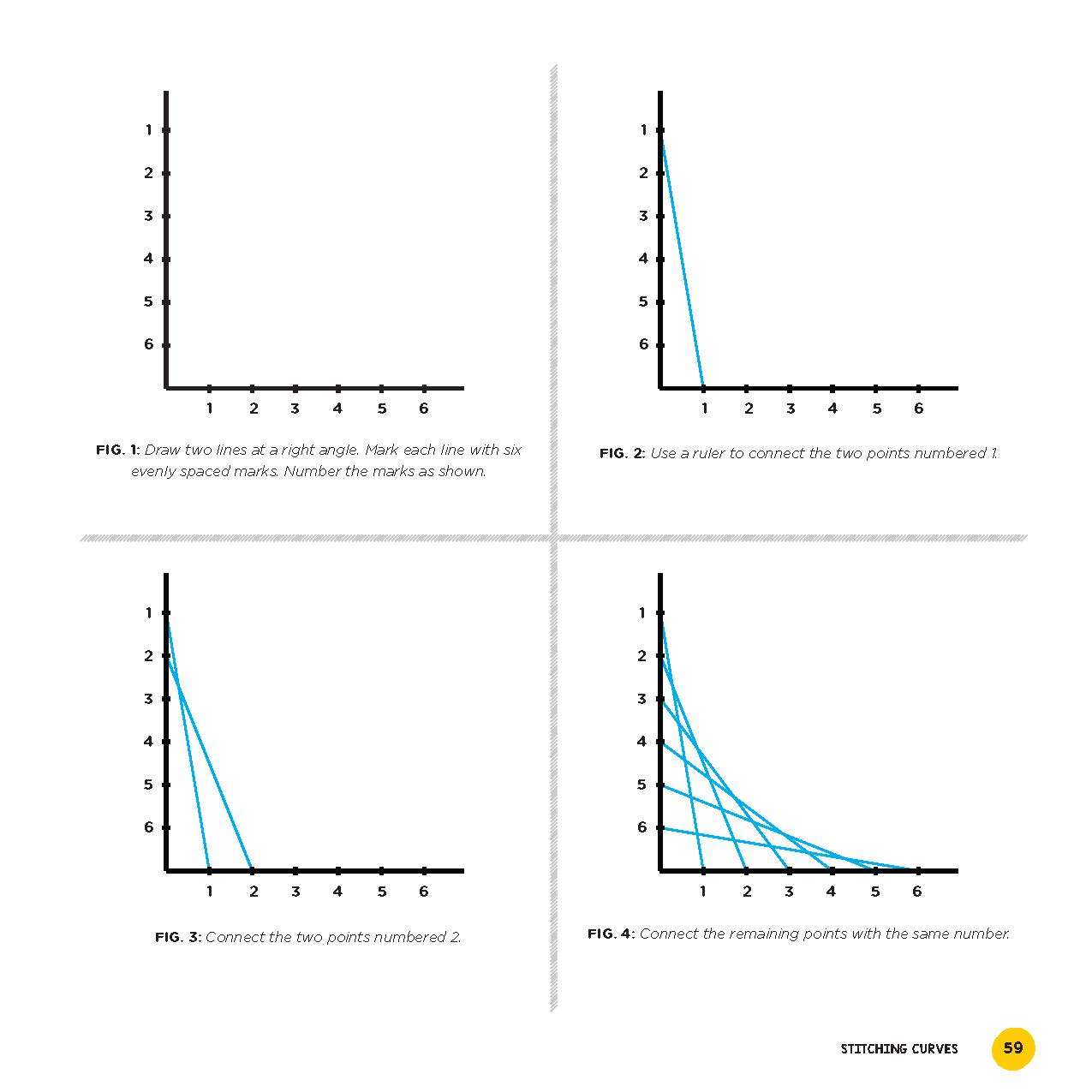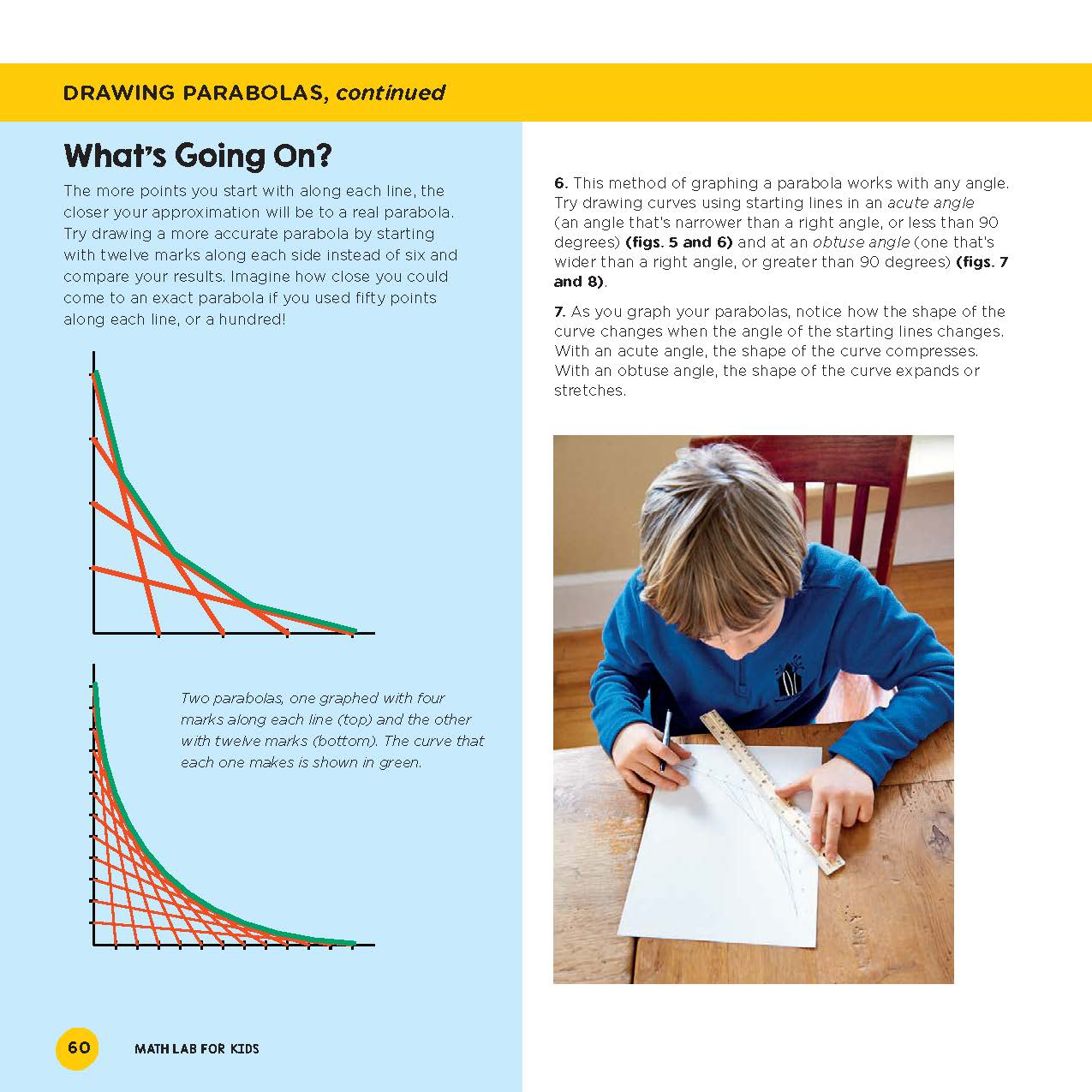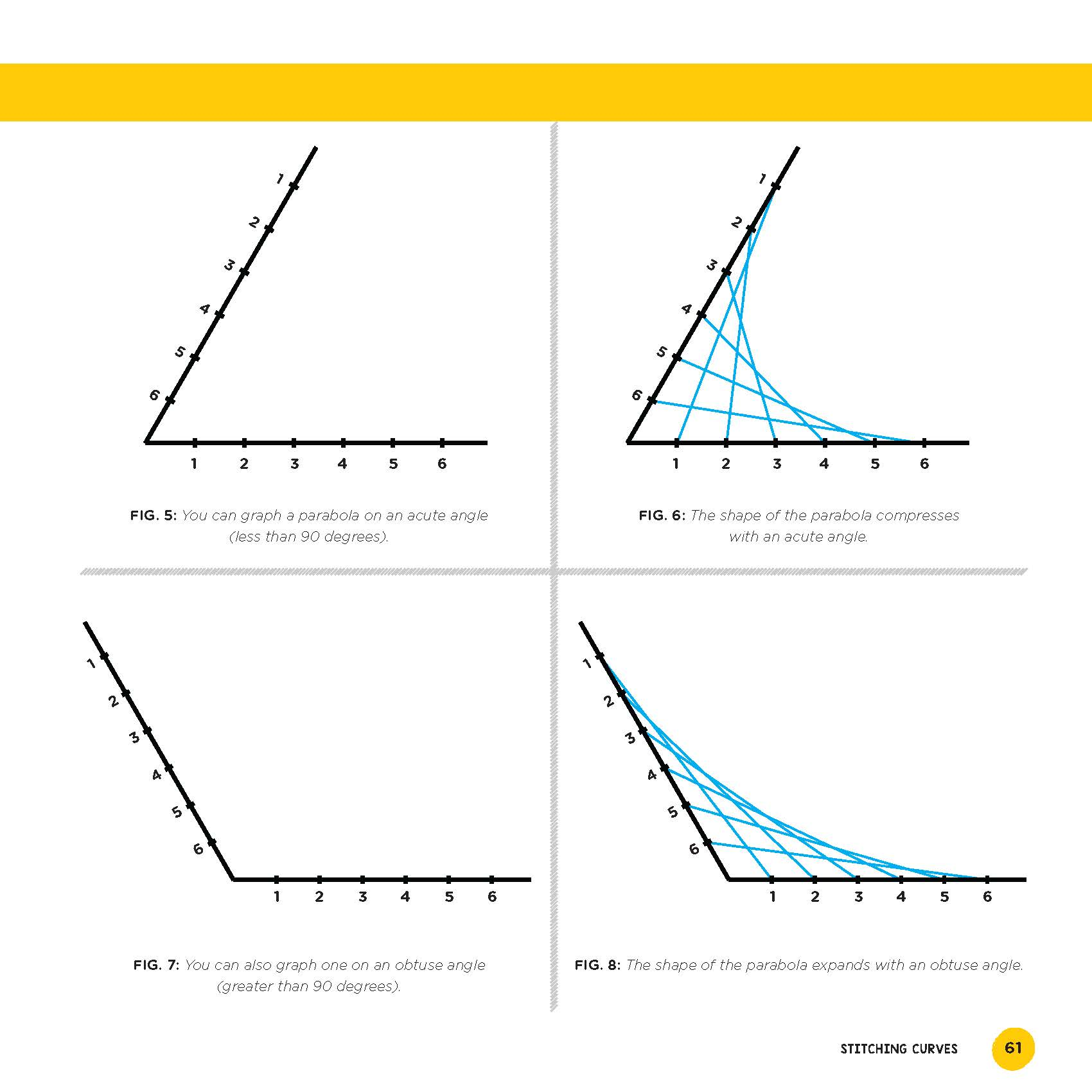Chapter 4, Stitching Curves, labs are:

• Lab 15: Drawing Parabolas
• Lab 16: Stitching Stars
• Lab 17: Creative Curves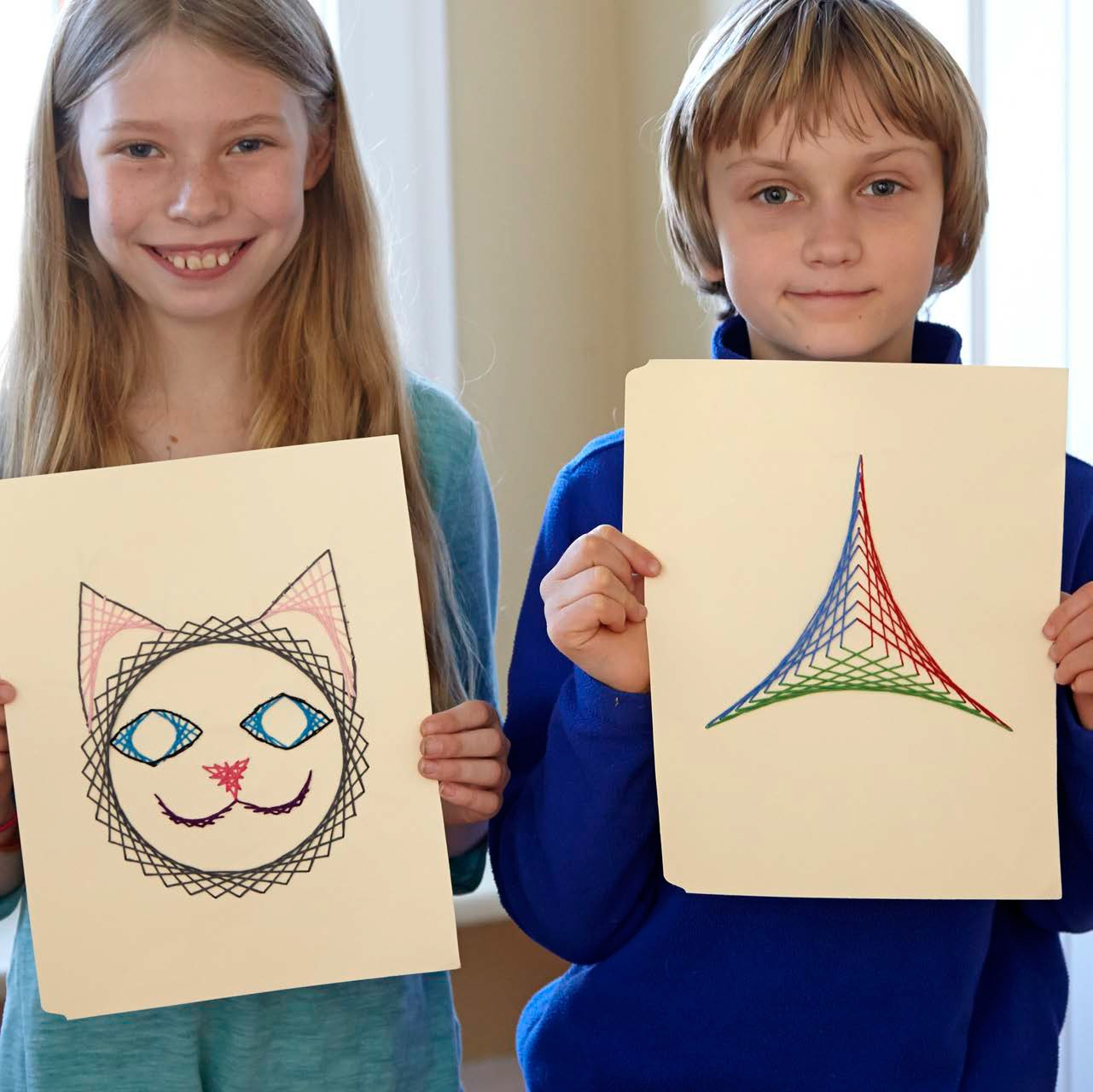# Lab 18: Draw a Sierpinski Triangle

Chapter 5, Fantastic Fractals introduces the beautiful world of Fractals. First we learn to draw fractals, then we build an enormous fractal, then we explore some interesting fractal properties.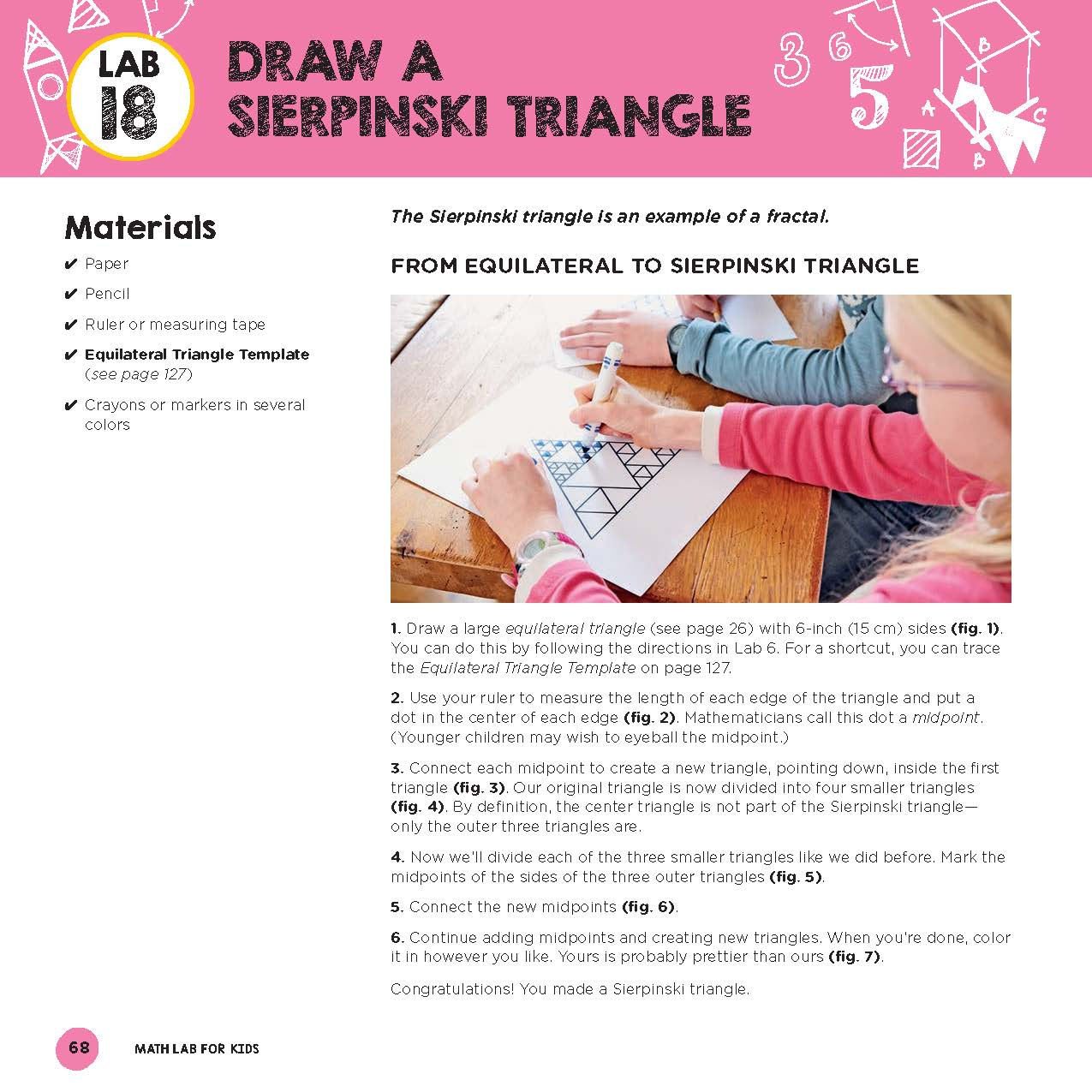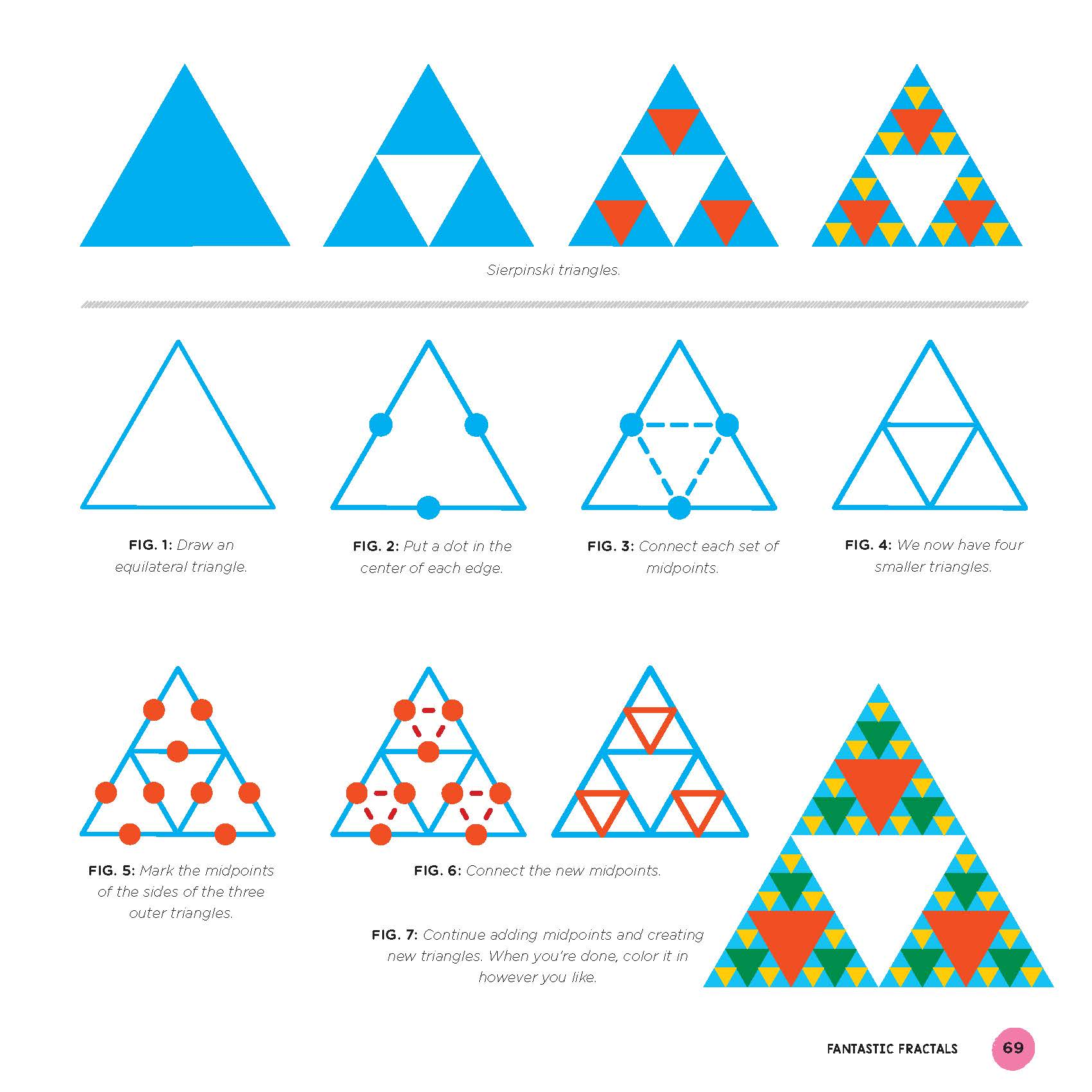Chapter 5, Fantastic Fractals, labs are:

• Lab 18: Draw a Sierpinski Triangle
• Lab 19: Build a Sierpinski Triangle
• Lab 20: Draw a Koch Snowflake
• Lab 21: Draw a Square Fractal Snowflake
• Lab 22: Explore the Koch Snowflake's Perimeter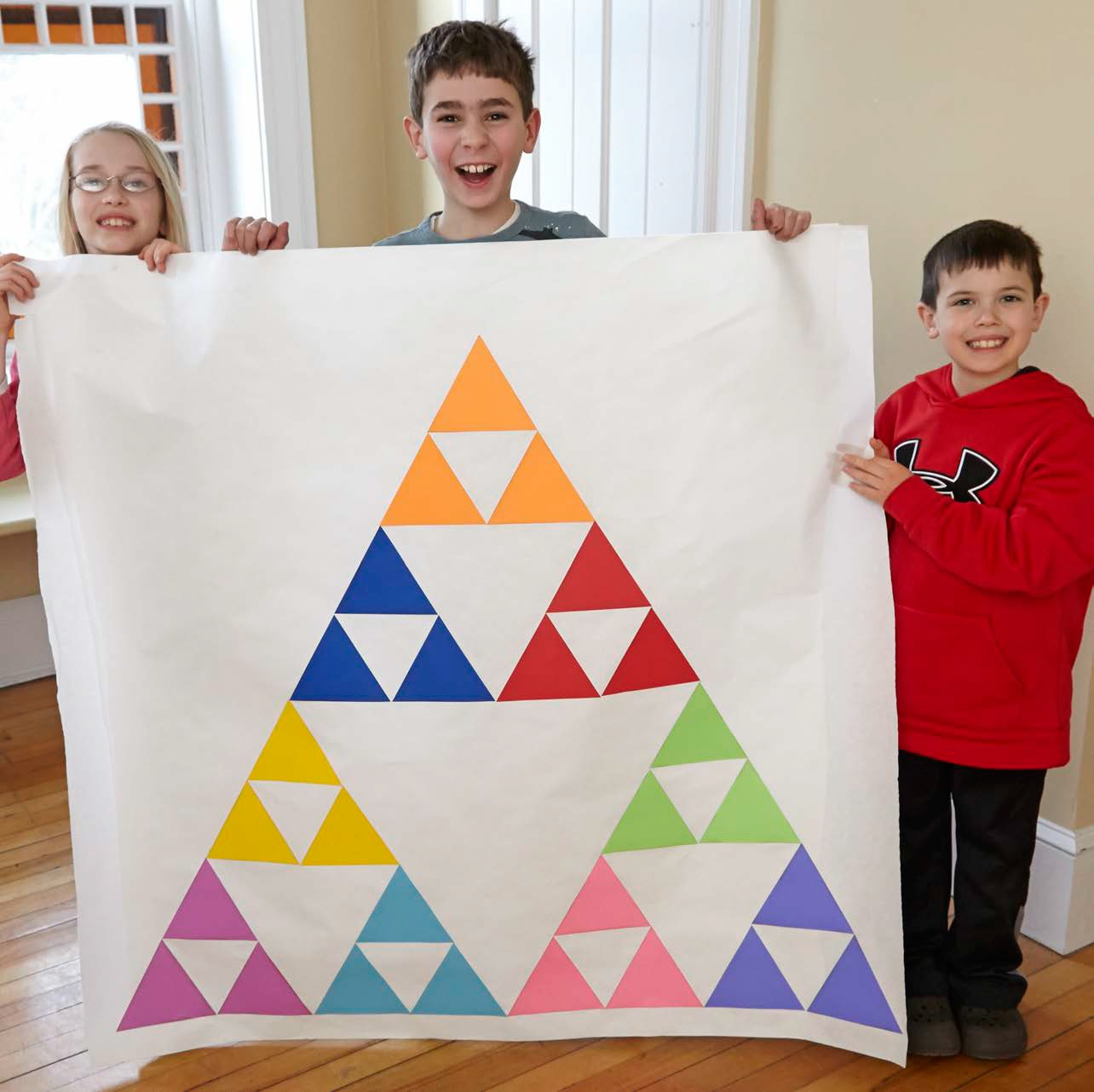# Lab 23: Tangram Basics

In Chapter 6, Terrific Tangrams, learn about this ancient Chinese puzzle where the same seven pieces are used to make many different shapes.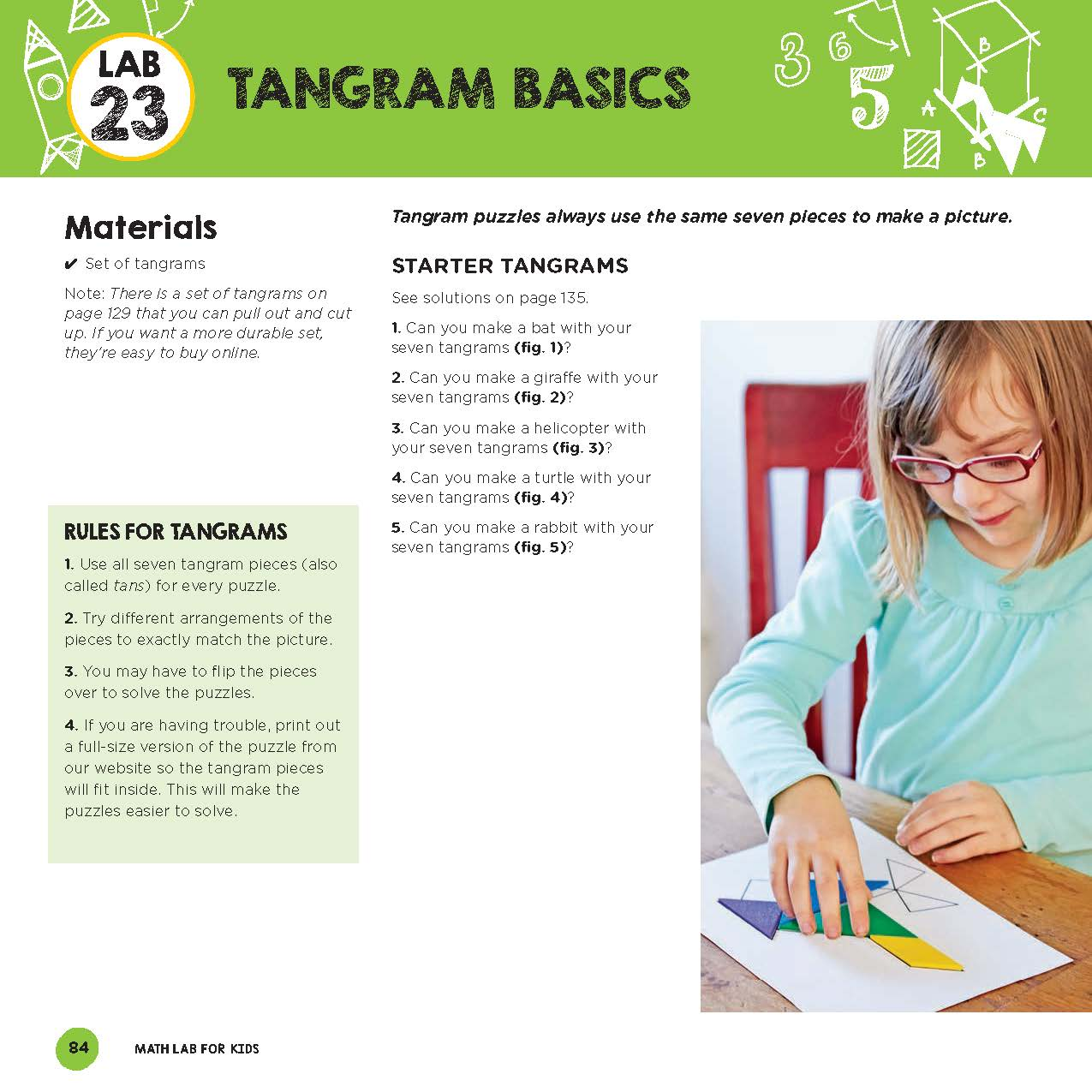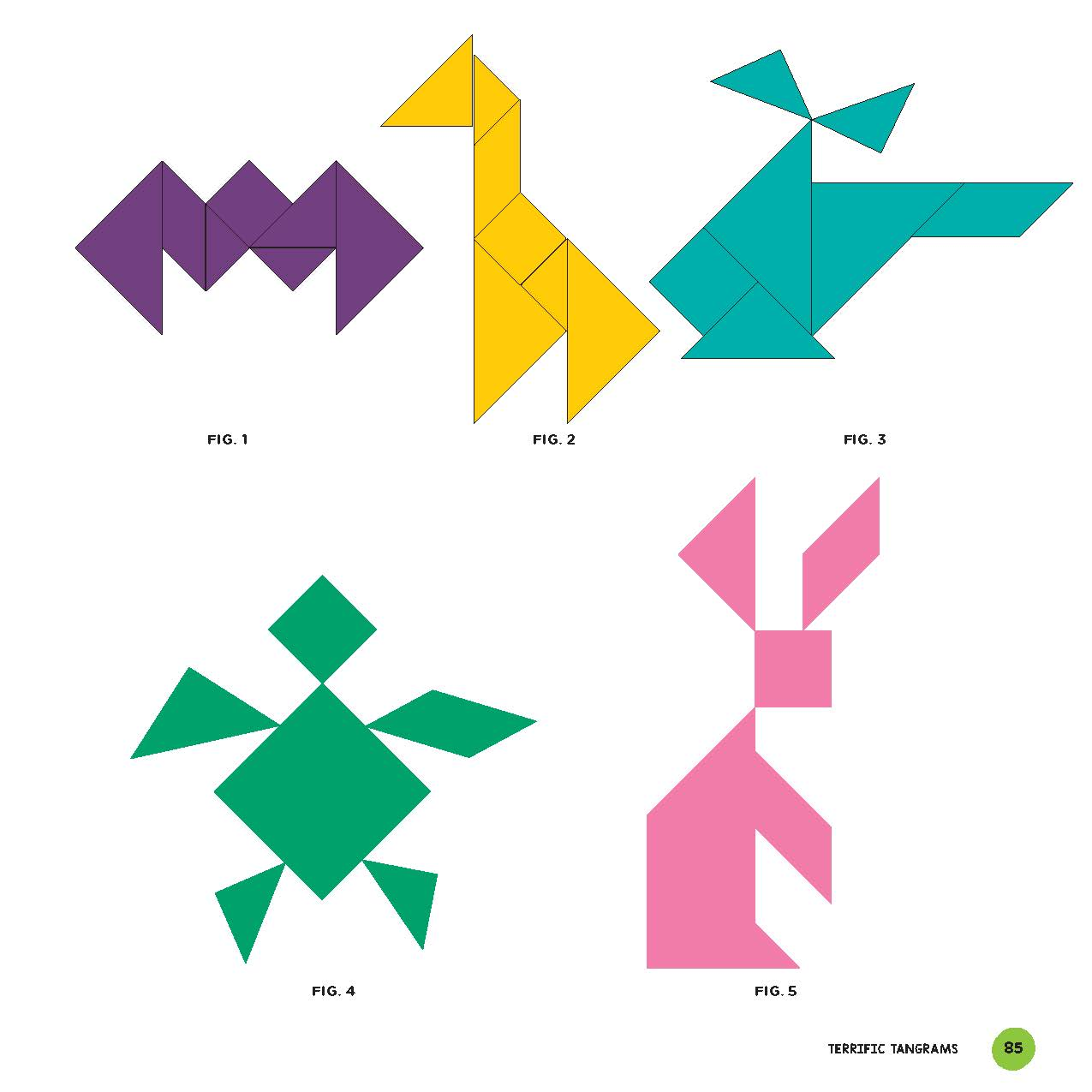Chapter 6, Terrific Tangrams, labs are:

• Lab 23: Tangram Basics
• Lab 24: Teaser Tangrams
• Lab 25: Tougher Tangrams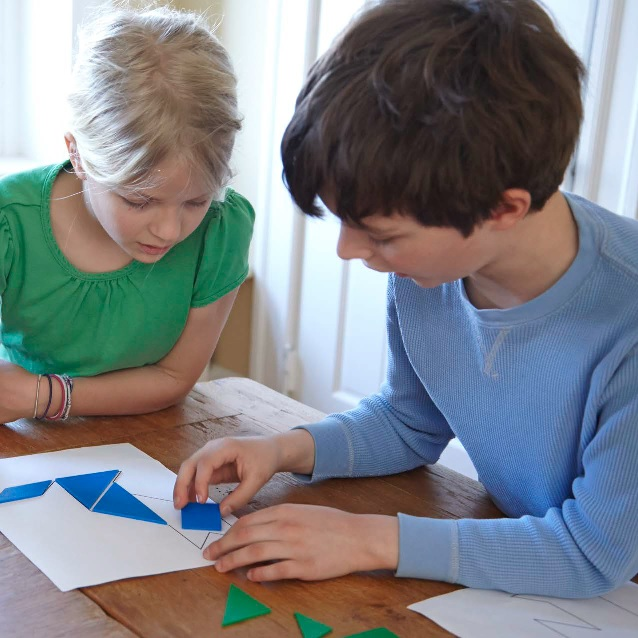# Lab 33: Eulerian Circuits

In Chapter 9, we learn about the Bridges of Königsberg, a puzzle that launched an entire field of mathematics, Graph Theory. We also introduce proof by induction and proof by contradiction.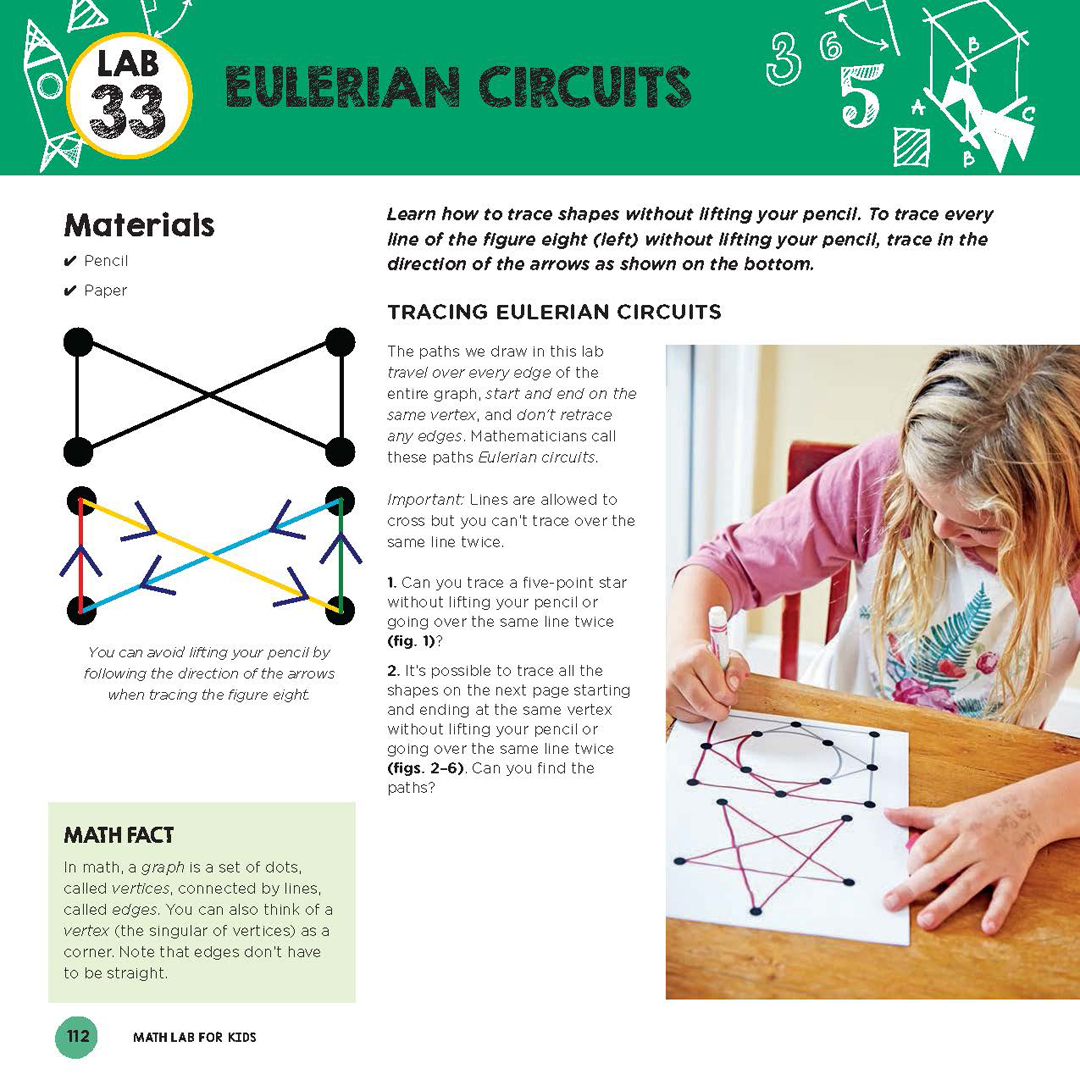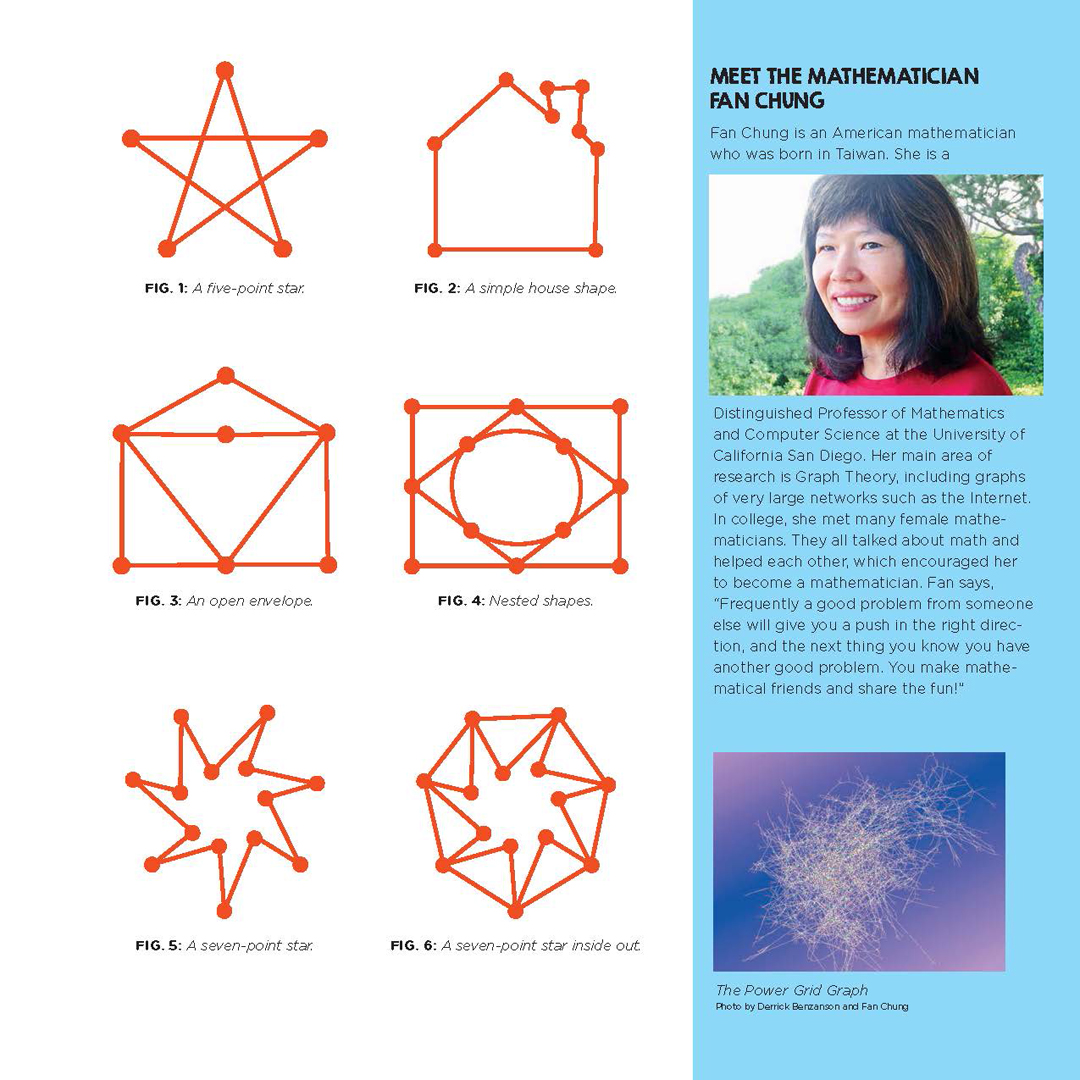Chapter 9, Graph Theory, labs are:

• Lab 33: Eulerian Circuits
• Lab 34: Secrets of Eulerian Circuits Revealed
• Lab 35: Bridges of Königsberg
• Lab 36: The Euler Characteristic
• Lab 37: A Proof About the Euler Characteristic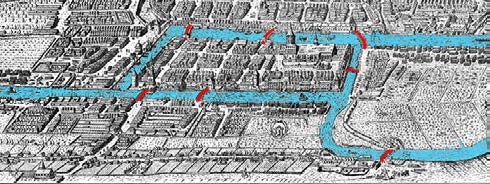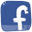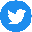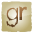Contact